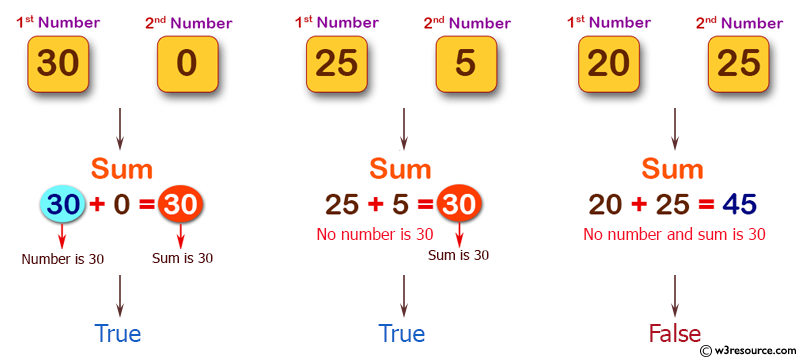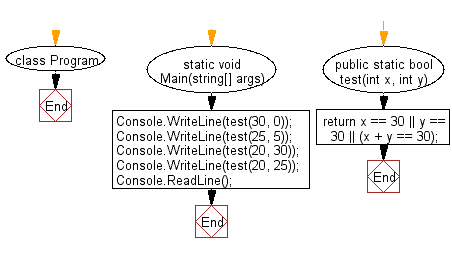﻿ C# - 2 integers, check one of them is 30 or their sum is 30# C# Sharp Basic Algorithm Exercises: Check two given integers, and return true if one of them is 30 or if their sum is 30

## C# Sharp Basic Algorithm: Exercise-3 with Solution

Write a C# Sharp program to check two given integers, and return true if one of them is 30 or if their sum is 30.

Pictorial Presentation:Sample Solution:

C# Sharp Code:

``````using System;
namespace exercises
{
class Program
{
static void Main(string[] args)
{
Console.WriteLine(test(30, 0));
Console.WriteLine(test(25, 5));
Console.WriteLine(test(20, 30));
Console.WriteLine(test(20, 25));
}

public static bool test(int x, int y)
{
return x == 30 || y == 30 || (x + y == 30);
}
}
}
```
```

Sample Output:

```True
True
True
False```

Flowchart:C# Sharp Code Editor:

Improve this sample solution and post your code through Disqus

What is the difficulty level of this exercise?

Test your Programming skills with w3resource's quiz.

﻿